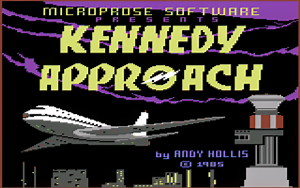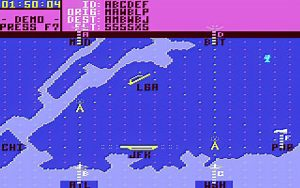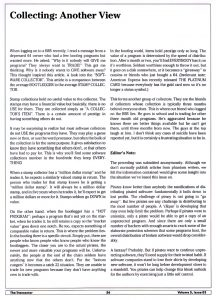# ELITE – Object ID Sheet

ELITE by FIREBIRD just seems to ADVANCE for 1985.
There was a few different versions with different copy protection.
Here in the USA one verions was GMA with extra tracks 36-40
Another version used Lenslock and a manual Symbol look up.

# Die Hard 2 – Code Sheet

Die Hard 2 used a code sheet for it’s copy protection.
The game will ask you for the arrival time of a flight and here they are:

ETAs for Die Hard 2 – Die Harder:

1. PC029 = 0509
2. BA123 = 1200
3. DA456 = 1330
4. BC789 = 1420
5. SA012 = 0955
6. TH256 = 0417
7. PC048 = 0258
8. MT369 = 0035
9. MC252 = 1939
10. LQ159 = 1830
11. ME357 = 1945
12. PV029 = 2036
13. AO146 = 1655
14. LZ358 = 2345
15. JW012 = 1409
16. JK356 = 0256
17. PA569 = 0652
18. AA143 = 1708
19. IS692 = 2120
20. JM713 = 1555
21. MW258 = 1618
22. PX016 = 0856
23. OT657 = 2234
24. AM359 = 0327
25. ST845 = 1102
26. VE379 = 0913
27. DA014 = 2345
28. IC391 = 1831
29. LS089 = 0600
30. JE473 = 2126
31. HJ193 = 0908
32. UI384 = 0548

# Kennedy Approach Code SheetKennedy Approach is such a fun game. Which I had spent hours and hours playing.Before you start the game you needed to enter a password.

It’s a shirt list, but here it is:

Kennedy Approach – Commodore 64
This game uses a code sheet:

1 – DME
2 – VOR
3 – ATC
4 – FAR
5 – IAP
6 – GCA
7 – FSS
8 – HAT
9 – VFR
10 – STAR
11 – SID
12 – JFR
13 – TDZ
14 – PAR
15 – NOB
16 – ELT

# Collecting: Another View

Back in the day me and my friends where Collectors. We downloaded and traded as many programs as we could. Did we use them? No. Did we play the games? Usually no. We just collected them. Maybe it’s an addiction? Were you a collector?